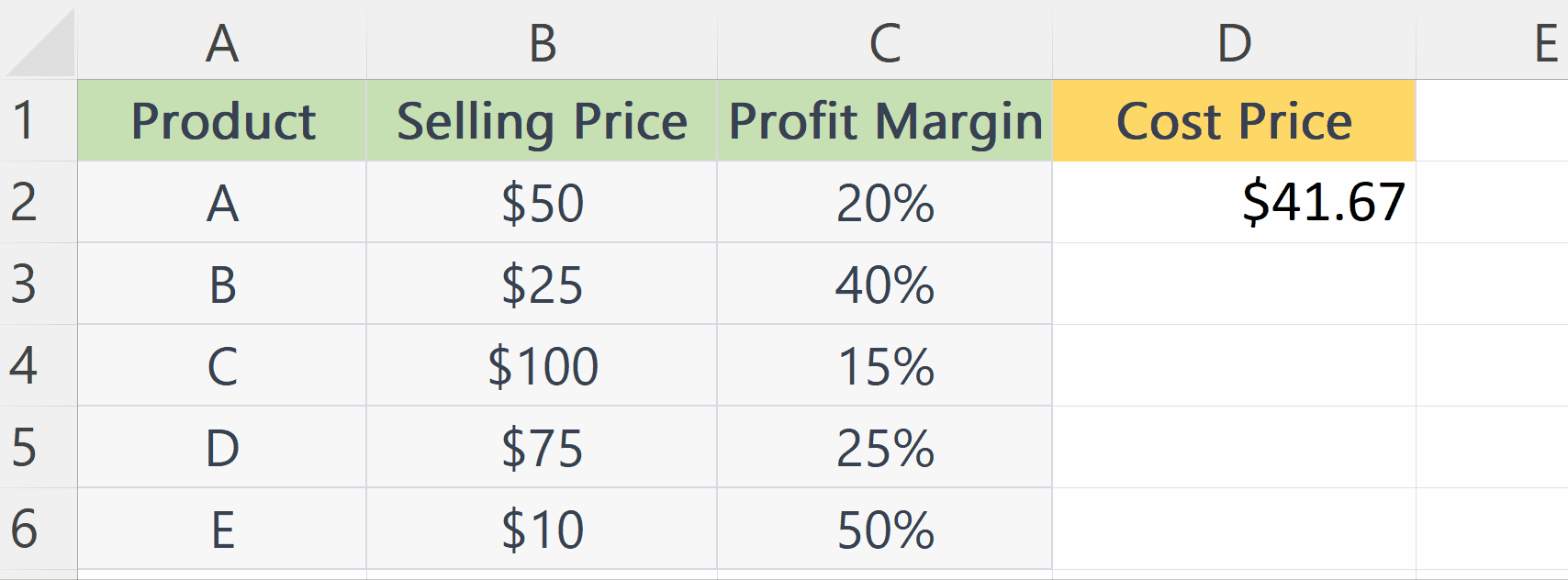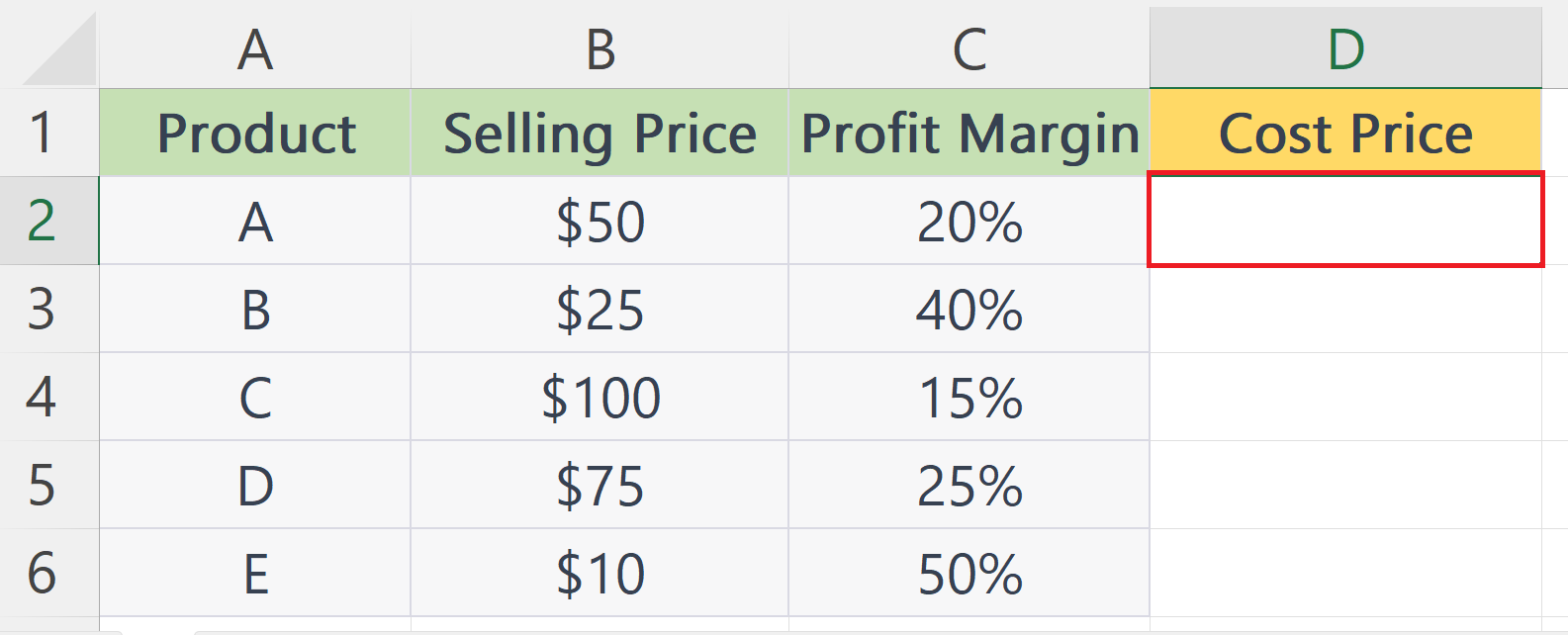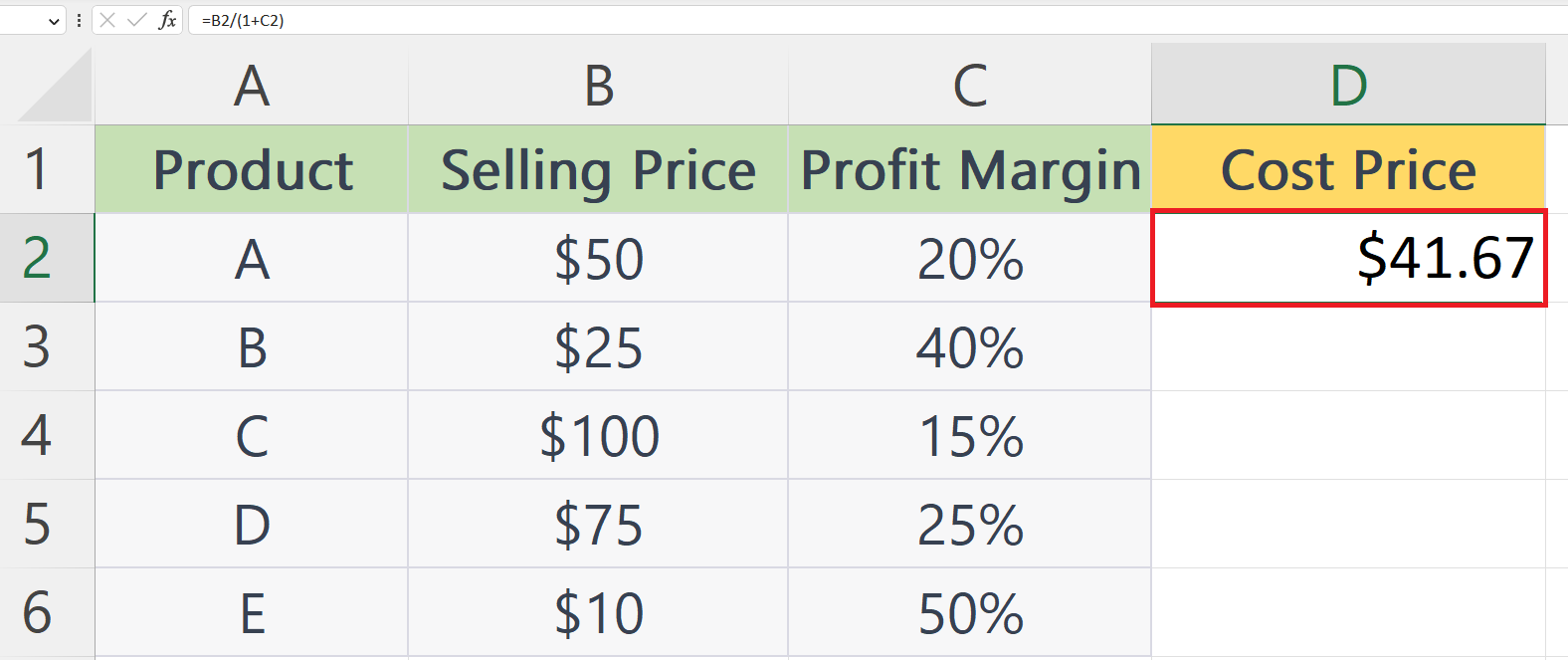# How to calculate cost price from selling price and margin in Microsoft Excel

In this tutorial, we will learn how to calculate the cost price from the selling price in Microsoft Excel. To calculate the cost price from the selling price and margin in Microsoft Excel, you can use the generic formula “cost price = selling price / (1 + margin)“. This formula takes into account the profit margin and helps you determine the original cost of the product. By using Excel’s formula feature, you can easily and accurately calculate the cost price for any given selling price and margin.

In the current data, the selling price and the profit margin of some products are listed. We will be calculating the cost price for each product.The cost price is the original price that a product was purchased or produced for before any additional costs or markup was added. Calculating the cost price from the selling price and margin in Microsoft Excel can be useful in determining the profitability of a product, and making informed pricing decisions.

### Step 1 – Select a Blank Cell– Select a blank cell where you want to calculate the cost price.

### Step 2 – Place an Equals Sign### Step 3 – Enter the Generic Formulae For Cost Price– Enter the generic formulae for cost price in the cell.
– The syntax of the formulae is:
Cost price = A2 / (1 + B2)
– Where A1 is the cell containing the selling price and B2 is the cell containing the profit margin.

### Step 4 – Press the Enter Key– Press the Enter key to calculate the cost price.

### Step 5 – Use the Autofill Feature to Calculate the Cost Price for Each Product– Use the Autofill feature to calculate the cost price for each product in the adjacent cells.